search
Search
Unlock 100+ guides
search toc
close
account_circle
Profile
exit_to_app
Sign out
search
keyboard_voice
close
Searching Tips
Search for a recipe:
"Creating a table in MySQL"
Search for an API documentation: "@append"
Search for code: "!dataframe"
Apply a tag filter: "#python"
Useful Shortcuts
/ to open search panel
Esc to close search panel
to navigate between search results
d to clear all current filters
Enter to expand content previewDoc SearchCode Search BetaSORRY NOTHING FOUND!
mic
Start speaking...Voice search is only supported in Safari and Chrome.
Shrink
Navigate to

# Comprehensive Guide on Subspace in Linear Algebra

schedule Aug 12, 2023
Last updated
local_offer
Linear Algebra
Tags
mode_heat
Master the mathematics behind data science with 100+ top-tier guides
Start your free 7-days trial now!
Definition.

# Subspace

Let $S$ be a subset of some vector space $V$. Then $S$ is a subspace if the following two conditions are satisfied:

• closed under addition - if $\boldsymbol{v}$ and $\boldsymbol{w}$ are in $S$, then $\boldsymbol{v}+\boldsymbol{w}$ is also in $S$.

• closed under scalar multiplication - if $\boldsymbol{v}$ is in $S$, then $k\boldsymbol{v}$ must be $S$, where $k$ is a scalar.

Example.

## Line passing through the origin

Consider the line $S$ in $\mathbb{R}^2$ that passes through the origin: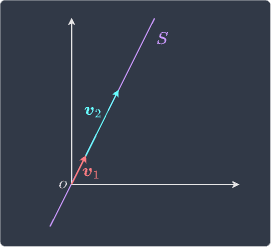This line $S$ is a subspace of $\mathbb{R}^2$ because adding any two vectors on the line, say $\boldsymbol{v}_1$ and $\boldsymbol{v}_2$ above, results in another vector on the line. This means that $S$ is closed under addition. Next, if we multiply any vector on the line, say $\boldsymbol{v}_1$, by any scalar $k$, then $k\boldsymbol{v}_1$ will also be on the line. This means that $S$ is closed under scalar multiplication. Since the two conditions are satisfied, $S$ is a subspace of $\mathbb{R}^2$.

The same idea holds for $\mathbb{R}^3$ - a line in $\mathbb{R}^3$ that passes through the origin is a subspace of $\mathbb{R}^3$.

Example.

## Line that does not pass through the origin

Consider the following line $S$ in $\mathbb{R}^2$ that does not pass through the origin: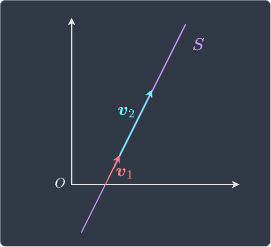Let $\boldsymbol{v}_1$ be any vector on $S$. Multiplying this vector by the scalar constant $0$ would give us the zero vector $\boldsymbol{0}$, which is not on $S$. This means that $S$ is not closed under scalar multiplication, and thus $S$ is not a subspace of $\mathbb{R}^2$.

Example.

## R2 is not subspace of R3

It is a common misconception that $\mathbb{R}^2$ is a subspace of $\mathbb{R}^3$. By definition, for $\mathbb{R}^2$ to be a subspace of $\mathbb{R}^3$, $\mathbb{R}^2$ must first be a subset of $\mathbb{R}^3$. This is not true because vectors in $\mathbb{R}^2$ have only two elements whereas vectors in $\mathbb{R}^3$ have three elements. This means that vectors in $\mathbb{R}^2$ do not belong in $\mathbb{R}^3$ - for instance:

$$\begin{pmatrix} 2\\3 \end{pmatrix}\notin\mathbb{R}^3$$

Therefore, $\mathbb{R}^2$ is not a subspace of $\mathbb{R}^3$.

Example.

## A plane passing through the origin

Consider the following plane:

$$2x+4y+3z=0$$

Show that this is a subspace of $\mathbb{R}^3$.

Solution. To show that the plane is a subspace of $\mathbb{R}^3$, we must check that the plane is closed under addition and scalar multiplication.

Suppose the following two generic vectors lie on the plane:

$$\boldsymbol{v}_1= \begin{pmatrix} x_1\\y_1\\z_1 \end{pmatrix},\;\;\;\;\; \boldsymbol{v}_2= \begin{pmatrix} x_2\\y_2\\z_2 \end{pmatrix}$$

Firstly, since these points are on the plane, we have that:

\begin{equation}\label{eq:M1IcX56LXG5HLrinvnh} \begin{aligned} 2x_1+4y_1+3z_1&=0\\ 2x_2+4y_2+3z_2&=0\\ \end{aligned} \end{equation}

Now, let's check whether or not the plane is closed under addition. The vector $\boldsymbol{v}_1+\boldsymbol{v}_2$ is:

\begin{equation}\label{eq:xWDCFePt8ckIu5VeeW0} \begin{aligned}[b] \boldsymbol{v}_1+ \boldsymbol{v}_2&= \begin{pmatrix} x_1\\y_1\\z_1 \end{pmatrix}+ \begin{pmatrix} x_2\\y_2\\z_2 \end{pmatrix}\\ &= \begin{pmatrix} x_1+x_2\\y_1+y_2\\z_1+z_2 \end{pmatrix} \end{aligned} \end{equation}

Let's substitute \eqref{eq:xWDCFePt8ckIu5VeeW0} into the equation of the plane to check whether this point is on the plane:

\begin{align*} 2(x_1+x_2)+4(y_1+y_2)+3(z_1+z_2) &=2x_1+2x_2+4y_1+4y_2+3z_1+3z_2\\ &=(2x_1+4y_1++3z_1)+(2x_2+4y_2+3z_2)\\ &=0 \end{align*}

The last step used \eqref{eq:M1IcX56LXG5HLrinvnh}. Therefore, this equation is closed under addition.

Now, we check whether or not the plane is closed under scalar multiplication. Let a point on the plane be represented by the following position vector:

$$\boldsymbol{v}= \begin{pmatrix} x\\y\\z \end{pmatrix}$$

Since this point lies on the plane, we have that:

$$\begin{equation}\label{eq:C2FeSwM2jZafDHhdC5g} 2x+4y+3z=0 \end{equation}$$

If $k$ is some scalar, then vector $k\boldsymbol{v}$ is:

$$k\boldsymbol{v}= \begin{pmatrix} kx\\ky\\kz \end{pmatrix}$$

Let's check if this point lies on the plane as well:

\begin{align*} 2(kx)+4(ky)+3(kz) &=2kx+4ky+3kz\\ &=k(2x+4y+3z)\\ &=0 \end{align*}

The last step used \eqref{eq:C2FeSwM2jZafDHhdC5g}. This means that the plane is also closed under scalar multiplication.

Because the plane is closed under addition and scalar multiplication, we conclude that the plane is a subspace of $\mathbb{R}^3$.

The plane is visualized below: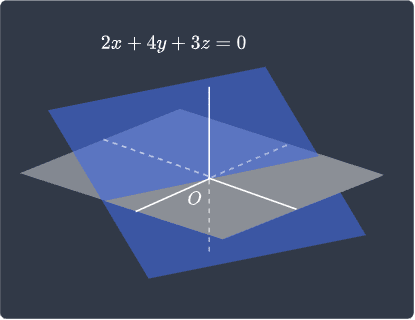As we can see, the plane cuts through the origin. In fact, for a plane to be a subspace of $\mathbb{R}^3$, the plane must pass through the origin for the same reason we covered in our previous examplelink - multiplying a scalar value of $0$ to any position vector will result in the zero vector! Therefore, if the plane does not pass through the origin, then the plane will not be closed under scalar multiplication and is therefore not a valid subspace.

Theorem.

# Spanning set of vectors is a subspace

If $\boldsymbol{v}_1$, $\boldsymbol{v}_2$, $\cdots$, $\boldsymbol{v}_p$ are in a vector space $V$, then $\mathrm{span}(\boldsymbol{v}_1, \boldsymbol{v}_2, \cdots, \boldsymbol{v}_p)$ is a subspace of $V$.

Proof. The span of a set of vectors is defined as the set of all possible linear combinations of the vectors, that is:

$$\mathrm{span}(\boldsymbol{v}_1,\boldsymbol{v}_2,\cdots,\boldsymbol{v}_n) = \{c_1\boldsymbol{v}_1+c_2\boldsymbol{v}_2+\cdots+c_n\boldsymbol{v}_n \;|\;c_1,c_2,\cdots,c_n\in\mathbb{R} \}$$

To prove that the span of the vectors is a subspace of $V$, we must show that the vectors in the span are closed under addition and also closed under scalar multiplication.

Let $\boldsymbol{v}$ and $\boldsymbol{w}$ be any two vectors in $\mathrm{span}(\boldsymbol{v}_1,\boldsymbol{v}_2,\cdots,\boldsymbol{v}_n)$. This means that $\boldsymbol{v}$ and $\boldsymbol{w}$ must be expressible using some linear combination of the vectors $\boldsymbol{v}_1$, $\boldsymbol{v}_2$, $\cdots$, $\boldsymbol{v}_n$ like so:

\begin{align*} \boldsymbol{v}&= c_1\boldsymbol{v}_1+c_2\boldsymbol{v}_2+\cdots+c_n\boldsymbol{v}_n\\ \boldsymbol{w}&= k_1\boldsymbol{v}_1+k_2\boldsymbol{v}_2+\cdots+k_n\boldsymbol{v}_n \end{align*}

The vector $\boldsymbol{v}+\boldsymbol{w}$ is:

\begin{align*} \boldsymbol{v}+\boldsymbol{w}&= (c_1+k_1)\boldsymbol{v}_1+ (c_2+k_2)\boldsymbol{v}_2+ \cdots+ (c_n+k_n)\boldsymbol{v}_n \end{align*}

Because $c_1+k_1$, $c_2+k_2$, $\cdots$, $c_n+k_n$ are also just constants, we have that $\boldsymbol{v}+\boldsymbol{w}$ is also expressible using some linear combination of the vectors. Therefore, the span of the vectors is closed under addition.

Now, consider any vector $\boldsymbol{v}$ again:

$$\boldsymbol{v}= c_1\boldsymbol{v}_1+c_2\boldsymbol{v}_2+\cdots+c_n\boldsymbol{v}_n$$

Multiplying $\boldsymbol{v}$ by any scalar constant $k$ gives:

\begin{align*} k\boldsymbol{v}&= k\big(c_1\boldsymbol{v}_1+c_2\boldsymbol{v}_2+\cdots+c_n\boldsymbol{v}_n\big)\\ &= (kc_1)\boldsymbol{v}_1+(kc_2)\boldsymbol{v}_2+\cdots+(kc_n)\boldsymbol{v}_n\\ \end{align*}

Here, $kc_1$, $kc_2$, $\cdots$, $kc_n$ are all constants, which means vector $k\boldsymbol{v}$ is can be expressed as a linear combination of the vectors. Therefore, the span of the vectors is also closed under scalar multiplication.

Because $\mathrm{span}(\boldsymbol{v}_1,\boldsymbol{v}_2,\cdots,\boldsymbol{v}_n)$ is closed under addition and also closed under scalar multiplication, the span is a subspace of $V$.

Example.

## Intuition behind spans in R2

Suppose we have a single position vector $\boldsymbol{v}$ like so: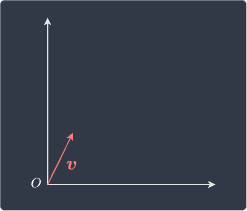The span of $\boldsymbol{v}$ is the set of all possible linear combinations of $\boldsymbol{v}$. Since there is only one vector $\boldsymbol{v}$, the linear combinations of $\boldsymbol{v}$ would be the scalar multiples of $\boldsymbol{v}$. We know multiplying a vector by a scalar has the effect of stretching/shrinking the vector while preserving its direction, so the linear combination of $\boldsymbol{v}$ traces out a line: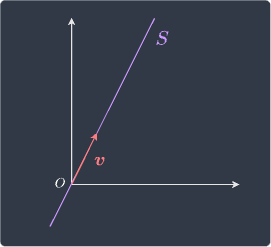We have already proven that the span of $\boldsymbol{v}$ must be a subspace of the vector space $\mathbb{R}^2$. This result agrees with the examplelink that any line $S$ that passes through the origin is a subspace of $\mathbb{R}^2$.

Example.

## Spanning set of three vectors

Suppose we have the following three vectors:

$$\boldsymbol{v}_1= \begin{pmatrix} 3\\2\\4 \end{pmatrix},\;\;\;\;\; \boldsymbol{v}_2= \begin{pmatrix} 1\\5\\2 \end{pmatrix},\;\;\;\;\; \boldsymbol{v}_3= \begin{pmatrix} 6\\1\\3 \end{pmatrix}$$

Consider the following sets of vectors:

• $S_1=\mathrm{span}(\boldsymbol{v}_1)$.

• $S_2=\mathrm{span}(\boldsymbol{v}_2)$.

• $S_3=\mathrm{span}(\boldsymbol{v}_1,\boldsymbol{v}_2)$.

• $S_4=\mathrm{span}(\boldsymbol{v}_1,\boldsymbol{v}_2,\boldsymbol{v}_3)$.

Since the vectors are three-dimensional, the vector space we are dealing with is $\mathbb{R}^3$. By theoremlink, all of these sets are subspaces of $\mathbb{R}^3$.

Theorem.

# Vectors are linearly independent if and only if the intersection of their subspaces contains only the zero vector

Let $\boldsymbol{w}_1$ be any vector in $W_1$ and $\boldsymbol{w}_2$ be any vector in $W_2$. The vectors $\boldsymbol{w}_1$ and $\boldsymbol{w}_2$ are linearly independent if and only if $W_1\cap{W_2}=\{\boldsymbol{0}\}$.

Solution. Suppose the following:

• $W_1$ has basis $\mathcal{B}_1=\{\boldsymbol{v}_1,\boldsymbol{v}_2,\cdots,\boldsymbol{v}_r\}$.

• $W_2$ has basis $\mathcal{B}_2=\{\boldsymbol{w}_1, \boldsymbol{w}_2, \cdots, \boldsymbol{w}_t \}$.

We first prove the forward proposition. Let $\boldsymbol{x}\in (W_1 \cap W_2)$, which means that $\boldsymbol{x}\in{W_1}$ and $\boldsymbol{x}\in{W_2}$. We can express $\boldsymbol{x}$ in terms of the basis vectors:

\begin{equation}\label{eq:QwBuvo84KeyGgChMIWT} \begin{aligned} \boldsymbol{x}&= c_1\boldsymbol{v}_1+ c_2\boldsymbol{v}_2+ \cdots+ c_r\boldsymbol{v}_r\\ \boldsymbol{x}&= d_1\boldsymbol{w}_1+ d_2\boldsymbol{w}_2+ \cdots+ d_t\boldsymbol{w}_t\\ \end{aligned} \end{equation}

Where $c_1$, $c_2$, $\cdots$, $c_r$ and $d_1$, $d_2$, $\cdots$, $d_t$ are scalar coefficients. Equating the two equations gives:

$$\begin{equation}\label{eq:bNZuWa9D03X8qOOqqAF} c_1\boldsymbol{v}_1+ c_2\boldsymbol{v}_2+ \cdots+ c_r\boldsymbol{v}_r= d_1\boldsymbol{w}_1+ d_2\boldsymbol{w}_2+ \cdots+ d_t\boldsymbol{w}_t \end{equation}$$

The left-hand side is a linear combination of the basis vectors $\mathcal{B}_1$ and the right-hand side is a linear combination of the basis vectors $\mathcal{B}_2$. Let $\boldsymbol{v}$ and $\boldsymbol{w}$ be defined as follows:

\begin{equation}\label{eq:WVF3G2mUDwvbAfPWKNV} \begin{aligned} \boldsymbol{v}&= c_1\boldsymbol{v}_1+ c_2\boldsymbol{v}_2+ \cdots+ c_r\boldsymbol{v}_r\\ \boldsymbol{w}&= d_1\boldsymbol{w}_1+ d_2\boldsymbol{w}_2+ \cdots+ d_t\boldsymbol{w}_t\\ \end{aligned} \end{equation}

We can now express \eqref{eq:bNZuWa9D03X8qOOqqAF} as:

$$\begin{equation}\label{eq:yPfYnl2hcIVEnH0DuzJ} \boldsymbol{v}=\boldsymbol{w} \end{equation}$$

Since we assume that $\boldsymbol{v}$ and $\boldsymbol{w}$ are linearly independent, the only way for \eqref{eq:yPfYnl2hcIVEnH0DuzJ} to hold is if $\boldsymbol{v}=\boldsymbol{w}=\boldsymbol{0}$. Let's take a moment to understand why. Imagine $\boldsymbol{v}\ne\boldsymbol{0}$ and $\boldsymbol{w}\ne\boldsymbol{0}$ - for instance:

$$\boldsymbol{v}= \begin{pmatrix} 3\\2 \end{pmatrix},\;\;\;\;\; \boldsymbol{w}= \begin{pmatrix} 3\\2 \end{pmatrix}$$

If this were true, then $\boldsymbol{v}$ and $\boldsymbol{w}$ is linearly dependent. This contradicts our assumption that $\boldsymbol{v}$ and $\boldsymbol{w}$ are linearly independent. Therefore, we can conclude that $\boldsymbol{v}=\boldsymbol{w}=\boldsymbol{0}$. With this, \eqref{eq:WVF3G2mUDwvbAfPWKNV} becomes:

\begin{equation}\label{eq:RWj6uQrh11AOSGaxOqL} \begin{aligned} \boldsymbol{0}&= c_1\boldsymbol{v}_1+ c_2\boldsymbol{v}_2+ \cdots+ c_r\boldsymbol{v}_r\\ \boldsymbol{0}&= d_1\boldsymbol{w}_1+ d_2\boldsymbol{w}_2+ \cdots+ d_t\boldsymbol{w}_t\\ \end{aligned} \end{equation}

Note the following:

• because $\boldsymbol{v}_1,\boldsymbol{v}_2,\cdots,\boldsymbol{v}_r$ are basis vectors, they are linearly independent by definitionlink. Therefore, we have that $c_1=c_2=\cdots=c_r=0$ by definitionlink of linear independence.

• similarly, because $\boldsymbol{w}_1$, $\boldsymbol{w}_2$, $\cdots$, $\boldsymbol{w}_t$ are basis vectors, they are linearly independent. Therefore, we have that $d_1=d_2=\cdots=d_t=0$.

This means that $\boldsymbol{x}=\boldsymbol{0}$ in \eqref{eq:QwBuvo84KeyGgChMIWT}. Therefore, we conclude that the intersection $W_1\cap{W_2}=\{\boldsymbol{0}\}$.

* * *

Let's now prove the converse. Assume $W_1\cap{W_2}=\{\boldsymbol{0}\}$. Our goal is to show that any vector $\boldsymbol{v}\in{W_1}$ and any vector $\boldsymbol{w}\in{W_2}$ are linearly independent. Consider the following equation:

$$\begin{equation}\label{eq:E4tfpVTACP5eCtvvGsv} c_1\boldsymbol{v}+ c_2\boldsymbol{w}=\boldsymbol{0} \end{equation}$$

Where $c_1$ and $c_2$ are some scalars. If we can show that $c_1=c_2=0$ for \eqref{eq:E4tfpVTACP5eCtvvGsv} to hold, then we can conclude that $\boldsymbol{v}$ and $\boldsymbol{w}$ are linearly independent by definitionlink.

Rearranging \eqref{eq:E4tfpVTACP5eCtvvGsv} gives:

$$\begin{equation}\label{eq:CQkreH9br0bH5UTmfC6} c_1\boldsymbol{v} =-c_2\boldsymbol{w} \end{equation}$$

Suppose there exists $c_1\ne{0}$ and $c_2\ne{0}$. Dividing both sides of \eqref{eq:CQkreH9br0bH5UTmfC6} by $c_1$ gives:

$$\boldsymbol{v} =-(c_2/c_1)\boldsymbol{w}$$

This contradicts our assumption that $W_1\cap{W_2}=\{\boldsymbol{0}\}$ since scalar multiples of $\boldsymbol{w}$ in $W_2$ also reside in $W_1$, which means that the intersection of $W_1$ and $W_2$ contains more than just the zero vector. Next suppose, $c_1=0$ and $c_2\ne0$. From \eqref{eq:CQkreH9br0bH5UTmfC6}, we have that $\boldsymbol{w}$ is the zero vector - but this cannot be true because $\boldsymbol{w}$ is any vector in $W_2$. Similarly, we cannot have the case where $c_1\ne0$ and $c_2=0$. Therefore, we conclude that $c_1=c_2=0$ for \eqref{eq:E4tfpVTACP5eCtvvGsv} to hold.

By definitionlink of linear independence, we conclude that $\boldsymbol{v}$ and $\boldsymbol{w}$ must be linearly independent. This completes the proof.

Theorem.

# Union of basis vectors is linearly independent if and only if the intersection of subspaces contains only the zero vector

Let $W_1$ and $W_2$ be subspaces of vector space $V$ and let $\mathcal{B}_1$ and $\mathcal{B}_2$ be the basis for $W_1$ and $W_2$ respectively. The union set $\mathcal{B}_1\cup{\mathcal{B}_2}$ is linearly independent if and only if $W_1\cap{W}_2=\{\boldsymbol{0}\}$.

Proof. The proof of this theorem is very similar to that of theoremlink. We first prove the forward proposition. Let the basis of $W_1$ and $W_2$ be:

\begin{align*} \mathcal{B}_1&= \left\{\boldsymbol{v}_1, \boldsymbol{v}_2, \cdots, \boldsymbol{v}_r\right\}\\ \mathcal{B}_2&= \{\boldsymbol{w}_1 \boldsymbol{w}_2, \cdots, \boldsymbol{w}_t\} \end{align*}

We assume that $\mathcal{B}_1\cup\mathcal{B}_2$ is linearly independent. We define any vector $\boldsymbol{x}\in(W_1\cap{W_2})$, which means that $\boldsymbol{x}\in{W}_1$ and $\boldsymbol{x}\in{W}_2$. Therefore, $\boldsymbol{x}$ can be expressed as linear combination of basis vectors in $\mathcal{B}_1$ and $\mathcal{B}_2$ like so:

\begin{equation}\label{eq:tsLmGuxUyHKIvFoqx6G} \begin{aligned} \boldsymbol{x}&= c_1\boldsymbol{v}_1+ c_2\boldsymbol{v}_2+ \cdots+ c_r\boldsymbol{v}_r\\ \boldsymbol{x}&= d_1\boldsymbol{w}_1+ d_2\boldsymbol{w}_2+ \cdots+ d_t\boldsymbol{w}_t\\ \end{aligned} \end{equation}

Where $c_1,c_2,\cdots,c_r$ and $d_1,d_2,\cdots,d_t$ are scalar coefficients. Equating the two equations gives:

\begin{align*} c_1\boldsymbol{v}_1+ c_2\boldsymbol{v}_2+ \cdots+ c_r\boldsymbol{v}_r&= d_1\boldsymbol{w}_1+ d_2\boldsymbol{w}_2+ \cdots+ d_t\boldsymbol{w}_t\\ c_1\boldsymbol{v}_1+ c_2\boldsymbol{v}_2+ \cdots+ c_r\boldsymbol{v}_r- (d_1\boldsymbol{w}_1+ d_2\boldsymbol{w}_2+ \cdots+ d_t\boldsymbol{w}_t)&= \boldsymbol{0}\\ c_1\boldsymbol{v}_1+ c_2\boldsymbol{v}_2+ \cdots+ c_r\boldsymbol{v}_r- d_1\boldsymbol{w}_1- d_2\boldsymbol{w}_2- \cdots- d_t\boldsymbol{w}_t&= \boldsymbol{0}\\ \end{align*}

Because we assume $\mathcal{B}_1\cup\mathcal{B}_2$ to be linearly independent, it follows from the definitionlink of linear independence that:

$$c_1=c_2\cdots=c_r= d_1=d_2\cdots=d_t=0$$

Substituting these scalar coefficients into \eqref{eq:tsLmGuxUyHKIvFoqx6G} gives:

$$\boldsymbol{x}=\boldsymbol{0}$$

Therefore, $W_1\cap{W_2}=\{\boldsymbol{0}\}$ and the forward proposition is proved.

* * *

Let's now prove the converse. We assume that $W_1\cap{W_2}=\{\boldsymbol{0}\}$. Let the basis for $W_1$ and $W_2$ be:

\begin{align*} \mathcal{B}_1&= \left\{\boldsymbol{v}_1, \boldsymbol{v}_2, \cdots, \boldsymbol{v}_r\right\}\\ \mathcal{B}_2&= \{\boldsymbol{w}_1 \boldsymbol{w}_2, \cdots, \boldsymbol{w}_t\} \end{align*}

Our goal is to show that the union $\mathcal{B}_1\cup\mathcal{B}_2$ is a linearly independent set. Consider the following equation:

$$\begin{equation}\label{eq:qEUblsj4l4Tg74Ihvrg} {\color{blue}c_1\boldsymbol{v}_1+ c_2\boldsymbol{v}_2+ \cdots+ c_r\boldsymbol{v}_r}+ {\color{red}d_1\boldsymbol{w}_1+ d_2\boldsymbol{w}_2+ \cdots+ d_t\boldsymbol{w}_t}= \boldsymbol{0}\\ \end{equation}$$

If we can show that $c_1=c_2=\cdots=c_r=d_1=d_2=\cdots=d_t=0$ for \eqref{eq:qEUblsj4l4Tg74Ihvrg} to hold, then the union set $\mathcal{B}_1\cup\mathcal{B}_2$ is linearly independent by definitionlink of linear independence. We define vectors $\boldsymbol{v}$ and $\boldsymbol{w}$ like so:

\begin{equation}\label{eq:TgmeT096Meo5JRybkGq} \begin{aligned} \boldsymbol{v}&= {\color{blue}c_1\boldsymbol{v}_1+ c_2\boldsymbol{v}_2+ \cdots+ c_r\boldsymbol{v}_r}\\ \boldsymbol{w}&= -({\color{red}d_1\boldsymbol{w}_1+ d_2\boldsymbol{w}_2+ \cdots+ d_t\boldsymbol{w}_t}) \end{aligned} \end{equation}

Using \eqref{eq:TgmeT096Meo5JRybkGq}, we can rewrite \eqref{eq:qEUblsj4l4Tg74Ihvrg} as:

$$\begin{equation}\label{eq:YUqkVieGjMURh7c3Blw} \boldsymbol{v}-\boldsymbol{w}= \boldsymbol{0} \end{equation}$$

Since $\boldsymbol{v}$ and $\boldsymbol{w}$ are linear combinations of the respective basis vectors, we have that $\boldsymbol{v}\in{W_1}$ and $\boldsymbol{w}\in{W_2}$. Rearranging \eqref{eq:YUqkVieGjMURh7c3Blw} gives:

$$\begin{equation}\label{eq:X082gta0SuZ9xdRQ279} \boldsymbol{v}= \boldsymbol{w} \end{equation}$$

Because $W_1\cap{W_2}=\{\boldsymbol{0}\}$, the only way for \eqref{eq:X082gta0SuZ9xdRQ279} to hold is if $\boldsymbol{v}=\boldsymbol{w}=\boldsymbol{0}$. Therefore, \eqref{eq:TgmeT096Meo5JRybkGq} is:

\begin{equation}\label{eq:Mz57HkhLyGXIMbFopcL} \begin{aligned} \boldsymbol{0}&= {\color{blue}c_1\boldsymbol{v}_1+ c_2\boldsymbol{v}_2+ \cdots+ c_r\boldsymbol{v}_r}\\ \boldsymbol{0}&= {\color{red}d_1\boldsymbol{w}_1+ d_2\boldsymbol{w}_2+ \cdots+ d_t\boldsymbol{w}_t} \end{aligned} \end{equation}

Note the following:

• because $\mathcal{B}_1=\{\boldsymbol{v}_1,\boldsymbol{v}_2,\cdots,\boldsymbol{v}_r\}$ is a basis for $W_1$, the set $\mathcal{B}_1$ is linearly independent by definitionlink.

• similarly, because $\mathcal{B}_2=\{\boldsymbol{w}_1,\boldsymbol{w}_2,\cdots,\boldsymbol{w}_t\}$ is a basis for $W_2$, the set $\mathcal{B}_2$ is linearly independent.

Therefore, by definitionlink of linear independence, we have that:

$$c_1=c_2=\cdots=c_r=d_1=d_2=\cdots=d_t=0$$

Because the scalar coefficients must be all zero for \eqref{eq:qEUblsj4l4Tg74Ihvrg} to hold, we conclude that $\mathcal{B}_1\cup\mathcal{B}_2$ is linearly independent. This completes the proof.

Theorem.

# Relationship between linear independence, subspace intersection and union of bases

Let $W_1$ and $W_2$ be subspaces of a vector space $V$ and let $\mathcal{B}_1$ and $\mathcal{B}_2$ be the basis for $W_1$ and $W_2$ respectively. The following statements are equivalent:

1. any vector $\boldsymbol{w}_1\in{W_1}$ and any vector $\boldsymbol{w}_2\in{W_2}$ are linearly independent.

2. $W_1\cap{W}_2=\{\boldsymbol{0}\}$ or $\mathrm{span}(\mathcal{B}_1)\cap \mathrm{span}(\mathcal{B}_2)=\{\boldsymbol{0}\}$.

3. the union set $\mathcal{B}_1\cup{\mathcal{B}_2}$ is linearly independent.

Proof. $(1)\Longleftrightarrow(2)$ is true by theoremlink. $(2)\Longleftrightarrow(3)$ is true by theoremlink. This means that:

$$(1)\Longleftrightarrow (2)\Longleftrightarrow (3)$$

This completes the proof.

Note that none of the statements in this theorem generalize to more vectors and subspaces. For instance, consider subspaces $W_1$, $W_2$ and $W_3$ with corresponding basis $\mathcal{B}_1$, $\mathcal{B}_2$ and $\mathcal{B}_3$ respectively. Now, assume $(1)$ that any vectors in $\boldsymbol{w}_1\in{W_1}$, $\boldsymbol{w}_2\in{W_2}$ and $\boldsymbol{w}_3\in{W_3}$ form a linearly independent set. Unfortunately, the union set $\mathcal{B}_1\cup \mathcal{B}_2\cup \mathcal{B}_3$ may not necessarily be linearly independent. For instance, consider the following case:

$$\mathcal{B}_1= \left\{\begin{pmatrix}1\\0\end{pmatrix}\right\}, \;\;\;\;\; \mathcal{B}_2= \left\{\begin{pmatrix}0\\1\end{pmatrix}\right\}, \;\;\;\;\; \mathcal{B}_3= \left\{\begin{pmatrix}1\\1\end{pmatrix}\right\}$$

Here, the union set is not linearly independent since we can construct the third basis vector using the first two basis vectors.

thumb_up
thumb_down
Comment
Citation
Ask a question or leave a feedback...
thumb_up
0
thumb_down
0
chat_bubble_outline
0
settings
Enjoy our search
Hit / to insta-search docs and recipes!